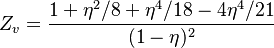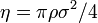# Difference between revisions of "Liu hard disk equation of state"$Z_v = \frac{1 + \eta^2/8 + \eta^4/18 - 4 \eta^4/21}{(1-\eta)^2}$
where the packing fraction is given by$\eta = \pi \rho \sigma^2 /4$ where$\sigma$ is the diameter of the disks.
$1 + \eta^2/8 + \eta^4/18 - 4 \eta^4/21}{(1-\eta)^2$